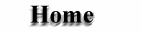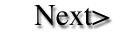## spctoolkit

by Donald J. Wheeler

Deciding which probability model is appropriate requires judgment that most students of statistics do not possess.

### What About Charts for Count Data?

Some data consist of counts rather than measurements. With count data, it has been tradition to use a theoretical approach for constructing control limits rather than an empirical approach for making measurements. The charts obtained by this theoretical approach have traditionally been known as "attribute charts." There are certain advantages and disadvantages of these charts.

Count data differ from measurement data in two ways. First, count data possess a certain irreducible discreteness that measurement data do not. Second, every count must have a known "area of opportunity" to be well-defined.

With measurement data, the discreteness of the values is a matter of choice. This is not the case with count data, which are based on the occurrence of discrete events (the so-called attributes). Count data always consist of integral values. This inherent discreteness is, therefore, a characteristic of the data and can be used in establishing control charts.

The area of opportunity for any given count defines the criteria by which the count must be interpreted. Before two counts may be compared, they must have corresponding (i.e., equally sized) areas of opportunity. If the areas of opportunity are not equally sized, then the counts must be converted into rates before they can be compared effectively. The conversion from counts to rates is accomplished by dividing each count by its own area of opportunity.

These two distinctive characteristics of count data have been used to justify different approaches for calculating the control limits of attribute charts. Hence, four control charts are commonly associated with count data-the np-chart, the p-chart, the c-chart and the u-chart. However, all four charts are for individual values.

The only difference between an XmR chart and an np-chart, p-chart, c-chart or u-chart is the way they measure dispersion. For any given set of count data, the X-chart and the four types of charts mentioned previously will show the same running records and central lines. The only difference between these charts will be the method used to compute the distance from the central line to the control limits.

The np-, p-, c- and u-charts all assume that the dispersion is a function of the location. That is, they assume that SD(X) is a function of MEAN(X). The application of the relationship between the parameters of a theoretical probability distribution must be justified by establishing a set of conditions. When the conditions are satisfied, the probability model is likely to approximate the behavior of the counts when the process displays a reasonable degree of statistical control.
Yet, deciding which probability model is appropriate requires judgment that most students of statistics do not possess. For example, the conditions for using a binomial probability model may be stated as:Binomial Condition 1: The area of opportunity for the count Y must consist of n distinct items.Binomial Condition 2: Each of the n distinct items must be classified as possessing, or not possessing, some attribute. This attribute is usually a type of nonconformance to specifications.Binomial Condition 3: Let p denote the probability that an item has the attribute being counted. The value of p must be the same for all n items in any one sample. While the chart checks if p changes from sample to sample, the value of p must be constant within each sample. Under the conditions, which are considered to be in a state of statistical control, it must be reasonable to assume that the value of p is the same for every sample.Binomial Condition 4: The likelihood that an item possessing the attribute will not be affected if the preceding item possessed the attribute. (This implies, for example, that nonconforming items do not naturally occur in clusters, and counts are independent of each other.)

If these four conditions apply to your data, then you may use the binomial model to compute an estimate of SD(X) directly from your estimate of MEAN(X). Or, you could simply place the counts (or proportions) on an XmR chart and estimate the dispersion from the moving range chart. You will obtain essentially the same chart either way.

Unlike attribute charts, XmR charts assume nothing about the relationship between the location and dispersion. It measures the location directly with the average, and it measures the dispersion directly with the moving ranges. Thus, while the np-, p-, c- and u-charts use theoretical limits, the XmR chart uses empirical limits. The only advantage of theoretical limits is that they include a larger number of degrees of freedom, which means that they stabilize more quickly.

If the theory is correct, and you use an XmR chart, the empirical limits will be similar to the theoretical limits. However, if the theory is wrong, the theoretical limits will be wrong, and the empirical limits will still be correct.

You can't go far wrong using an XmR chart with count data, and it is generally easier to work with empirical limits than to verify the conditions for a theoretical model.